Next: 4.5 Implications of Delayed Up: 4 Muonium formation in Previous: 4.3.2 Solid Nitrogen in

# 4.4 Delayed Muonium Formation

Muonium formation involving electrons originating in the radiolysis track depends on the transport of these electrons to the muon under the influence of their mutual Coulomb attraction and any external fields. The associated time-of-flight can be large enough to have an observable effect on timescales accessible by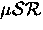if the initial separation is great enough.

While a muon awaits the arrival of an electron its spin will precess in an external transverse magnetic field at the muon Larmor frequency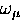, and if it decays during this time it will contribute to the diamagnetic signal. Those muons that do not decay while in a diamagnetic state will, upon forming muonium, begin to precess in the opposite sense at the characteristic frequencies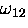and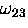, possibly contributing to the muonium signal instead. (A complete discussion of the muonium signal is given in Appendix A. In weak fields we may characterize the muonium signal by the average frequency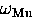.) The elapsed time between muons entering the sample and the arrival of electrons at the muons will have some distribution, so the coherent diamagnetic polarization will be converted to muonium polarization over a range of times, with a corresponding distribution in the apparent initial phase of the muonium precession. Sinceis very small compared to the muonium precession frequencies, the dephasing is almost entirely due to precession in the higher frequency final state starting at different times. The conversion of coherent muon polarization to partially dephased muonium polarization results in a measureable loss of asymmetry whenever the formation time is comparable to or larger than the muonium precession period. Delayed muonium formation is experimentally observable in principle in the shape of the diamagnetic relaxation function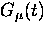, but it is more easily observed in the resulting muonium amplitude and phase after all Mu formation is complete. A stronger magnetic field results in proportionally more dephasing and therefore a reduced polarization of the delayed part of the muonium ensemble. One can thus estimate the characteristic time of muonium formation from the magnetic field dependence of the amplitude of the muonium signal.

At any time the instantaneous polarization is the vector sum of all muon spins present including diamagnetic, delayed and prompt muonium. If the distribution of formation times is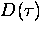[the probability of muonium formation between times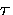and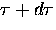is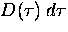] then the total polarization at time t is

in which f0 is the prompt muonium fraction. For clarity here we are ignoring the splitting of the muonium signal that would be apparent in transverse fields greater than about 10 G. In fitting the data, one must replace the single muonium signal above with the usual sum of two components at frequenciesand.The first term in Eq. (4.1) is the coherent diamagnetic polarization remaining at time t. The second term is the sum over all phases of the accumulated delayed muonium ensemble. The third term is the prompt muonium polarization.

After all muonium formation is complete, the resulting muonium precesses at the same frequency and undergoes spin relaxation according to the same function as the prompt muonium fraction. In this way the long-time muonium ensemble can be characterized by a single amplitude and phase. Figure 4.14 shows the asymmetry of the muonium ensemble (the total muonium signal) in liquid nitrogen at T=75 K in various transverse magnetic fields, obtained by fitting thespectra starting at t=50 ns. The solid line is the empirical maximum asymmetry A0 multiplied by the theoretical polarization in Eq. (4.1), and in which the distribution of formation times is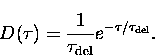[Although it is possible in principle to obtain,which may be a complicated function of the spatial distribution of electrons, the present data are not sufficient to reveal the real shape of D(t).] The characteristic formation time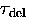estimated in this way was 14(4) ns and the muonium asymmetry (in the limit of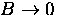) resulting from delayed formation was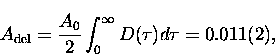while prompt muonium contributed A0 f0 = 0.038(2). From this we conclude that delayed formation accounts for at least 22% of the muonium in liquid nitrogen. (Muonium formed by the delayed'' process within a time very short in comparison to the precession period is indistinguishable from the prompt hot muonium fraction, so the delayed'' fraction could be larger.)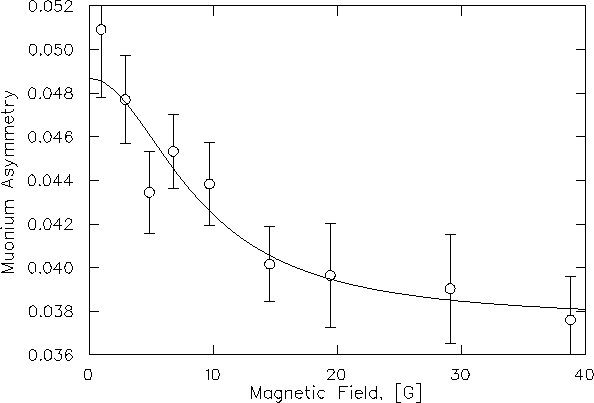Similar experiments on solid nitrogen and rare gas solids have not shown magnetic field dependence of asymmetries, presumably because the time of flight is too short due to greater electron mobility and/or reduced distance.

If the electrons were initially much further away from the muon than the width of their spatial distribution, then the maximum muonium formation rate could be displaced from t=0 by an amount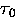.In this case, if the prompt muonium had time to precess one or more full revolutions while the electrons were diffusing to the muon, the total muonium polarization would be a periodic function of magnetic field. This would yield a direct measurement of the formation time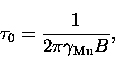where B is the period in magnetic field. Such convincing evidence of delayed muonium formation has not yet been observed, but is possible in principle.Next: 4.5 Implications of Delayed Up: 4 Muonium formation in Previous: 4.3.2 Solid Nitrogen in